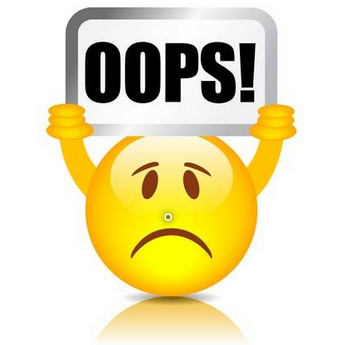### Consecutive Prime Sum

#### Description

Some prime numbers can be expressed as a sum of other consecutive prime numbers.
For example
5 = 2 + 3,
17 = 2 + 3 + 5 + 7,
41=2+3+5+7+11+13.
Your task is to find out how many prime numbers which satisfy this property are present in the range 3 to N subject to a constraint that summation should always start with number 2.
Write code to find out the number of prime numbers that satisfy the above-mentioned property in a given range.

#### Input Format

First line contains a number N

#### Output Format

Print the total number of all such prime numbers which are less than or equal to N.

Example 1:

Input
`20`
Output
`2`
Example 2:

Input
`10`
Output
`1`
Example 3:

Input
`50`
Output
`3`### Approach

Store all the prime Numbers upto n in an array.
Iterate a loop and add the prime numbers to a variable.
In the loop use binary search to search the sum is there in primes array or not.
If present in the array increase a count value.
Finally return the count.

Note :

Let us know if you can come up with a better approach, mail us at support@theinquisitive.in Your approach will be reviewed and posted with credits to you.